# Bayesian

Least square method: deciding model parameters by minimizing RSS (residual sum of squares)
ML (maximum likelihood) estimation: estimating model parameters by finding the parameter values that maximize the likelihood of making the observations given the parameters
MAP (maximum a posteriori) estimation

## Bayes’ Theorem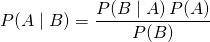where A and B are events and P(B) ≠ 0.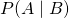: conditional probability of event A given that B is true.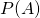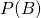: probability of event A, probability of event B without regarding each other.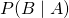: conditional probability of event A given that B is true.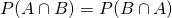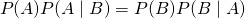### example

Suppose that a probability of having disease A is 0.5%.
And suppose that a test is 99% sensitive (true positive rate), 95% specific (true negative rate).
If you’re detected as positive by the test. What is the probability that you have disease A?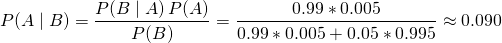Even if you’re diagnosed as positive, the probability of having disease is only around 9%

### Bayesian inference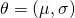: parameters of probability distribution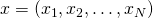: observed data (fixed)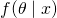: posterior probability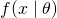: likelihood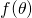: prior probability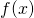: marginal likelihood or normalization constant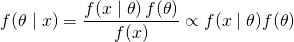Try to find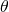which maximizeshttp://mlss.tuebingen.mpg.de/2015/slides/ghahramani/lect1bayes.pdf

http://hosho.ees.hokudai.ac.jp/~kubo/stat/2010/Qdai/b/kuboQ2010b.pdf